# 5.8 Modeling using variation  (Page 7/14)

 Page 7 / 14

## Dividing Polynomials

For the following exercises, use long division to find the quotient and remainder.

$\frac{{x}^{3}-2{x}^{2}+4x+4}{x-2}$

$\text{\hspace{0.17em}}{x}^{2}+4\text{\hspace{0.17em}}$ with remainder 12

$\frac{3{x}^{4}-4{x}^{2}+4x+8}{x+1}$

For the following exercises, use synthetic division to find the quotient. If the divisor is a factor, then write the factored form.

$\frac{{x}^{3}-2{x}^{2}+5x-1}{x+3}$

${x}^{2}-5x+20-\frac{61}{x+3}$

$\frac{{x}^{3}+4x+10}{x-3}$

$\frac{2{x}^{3}+6{x}^{2}-11x-12}{x+4}$

$2{x}^{2}-2x-3$ , so factored form is $\left(x+4\right)\left(2{x}^{2}-2x-3\right)$

$\frac{3{x}^{4}+3{x}^{3}+2x+2}{x+1}$

## Zeros of Polynomial Functions

For the following exercises, use the Rational Zero Theorem to help you solve the polynomial equation.

$2{x}^{3}-3{x}^{2}-18x-8=0$

$3{x}^{3}+11{x}^{2}+8x-4=0$

$2{x}^{4}-17{x}^{3}+46{x}^{2}-43x+12=0$

$4{x}^{4}+8{x}^{3}+19{x}^{2}+32x+12=0$

For the following exercises, use Descartes’ Rule of Signs to find the possible number of positive and negative solutions.

${x}^{3}-3{x}^{2}-2x+4=0$

0 or 2 positive, 1 negative

$2{x}^{4}-{x}^{3}+4{x}^{2}-5x+1=0$

## Rational Functions

For the following exercises, find the intercepts and the vertical and horizontal asymptotes, and then use them to sketch a graph of the function.

$f\left(x\right)=\frac{x+2}{x-5}$

Intercepts $\left(–2,0\right)\text{and}\left(0,-\frac{2}{5}\right)$ , Asymptotes $\text{\hspace{0.17em}}x=5\text{\hspace{0.17em}}$ and $\text{\hspace{0.17em}}y=1.$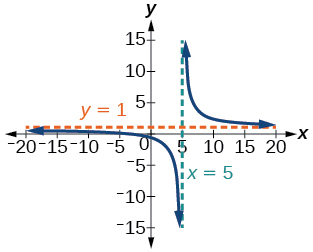$f\left(x\right)=\frac{{x}^{2}+1}{{x}^{2}-4}$

$f\left(x\right)=\frac{3{x}^{2}-27}{{x}^{2}+x-2}$

Intercepts (3, 0), (-3, 0), and $\text{\hspace{0.17em}}\left(0,\frac{27}{2}\right)\text{\hspace{0.17em}}$ , Asymptotes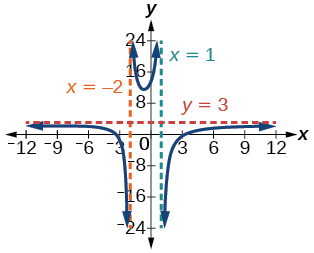$f\left(x\right)=\frac{x+2}{{x}^{2}-9}$

For the following exercises, find the slant asymptote.

$f\left(x\right)=\frac{{x}^{2}-1}{x+2}$

$f\left(x\right)=\frac{2{x}^{3}-{x}^{2}+4}{{x}^{2}+1}$

## Inverses and Radical Functions

For the following exercises, find the inverse of the function with the domain given.

$f\left(x\right)={\left(x-2\right)}^{2},\text{\hspace{0.17em}}x\ge 2$

${f}^{-1}\left(x\right)=\sqrt{x}+2$

$f\left(x\right)={\left(x+4\right)}^{2}-3,\text{\hspace{0.17em}}x\ge -4$

$f\left(x\right)={x}^{2}+6x-2,\text{\hspace{0.17em}}x\ge -3$

${f}^{-1}\left(x\right)=\sqrt{x+11}-3$

$f\left(x\right)=2{x}^{3}-3$

$f\left(x\right)=\sqrt{4x+5}-3$

${f}^{-1}\left(x\right)=\frac{{\left(x+3\right)}^{2}-5}{4},\text{\hspace{0.17em}}x\ge -3$

$f\left(x\right)=\frac{x-3}{2x+1}$

## Modeling Using Variation

For the following exercises, find the unknown value.

$\text{\hspace{0.17em}}y\text{\hspace{0.17em}}$ varies directly as the square of $\text{\hspace{0.17em}}x.\text{\hspace{0.17em}}$ If when find $\text{\hspace{0.17em}}y\text{\hspace{0.17em}}$ if $\text{\hspace{0.17em}}x=4.$

$y=64$

$\text{\hspace{0.17em}}y\text{\hspace{0.17em}}$ varies inversely as the square root of $\text{\hspace{0.17em}}x\text{\hspace{0.17em}}$ If when find $\text{\hspace{0.17em}}y\text{\hspace{0.17em}}$ if $\text{\hspace{0.17em}}x=4.$

$\text{\hspace{0.17em}}y\text{\hspace{0.17em}}$ varies jointly as the cube of $\text{\hspace{0.17em}}x\text{\hspace{0.17em}}$ and as $\text{\hspace{0.17em}}z.\text{\hspace{0.17em}}$ If when $\text{\hspace{0.17em}}x=1\text{\hspace{0.17em}}$ and $\text{\hspace{0.17em}}z=2,\text{\hspace{0.17em}}$ $y=6,\text{\hspace{0.17em}}$ find $\text{\hspace{0.17em}}y\text{\hspace{0.17em}}$ if $\text{\hspace{0.17em}}x=2\text{\hspace{0.17em}}$ and $\text{\hspace{0.17em}}z=3.$

$\text{\hspace{0.17em}}y\text{\hspace{0.17em}}$ varies jointly as $\text{\hspace{0.17em}}x\text{\hspace{0.17em}}$ and the square of $\text{\hspace{0.17em}}z\text{\hspace{0.17em}}$ and inversely as the cube of $\text{\hspace{0.17em}}w.\text{\hspace{0.17em}}$ If when $\text{\hspace{0.17em}}x=3,\text{\hspace{0.17em}}$ $z=4,\text{\hspace{0.17em}}$ and $\text{\hspace{0.17em}}w=2,\text{\hspace{0.17em}}$ $y=48,\text{\hspace{0.17em}}$ find $\text{\hspace{0.17em}}y\text{\hspace{0.17em}}$ if $\text{\hspace{0.17em}}x=4,\text{\hspace{0.17em}}$ $z=5,\text{\hspace{0.17em}}$ and $\text{\hspace{0.17em}}w=3.$

For the following exercises, solve the application problem.

The weight of an object above the surface of the earth varies inversely with the distance from the center of the earth. If a person weighs 150 pounds when he is on the surface of the earth (3,960 miles from center), find the weight of the person if he is 20 miles above the surface.

148.5 pounds

The volume $\text{\hspace{0.17em}}V\text{\hspace{0.17em}}$ of an ideal gas varies directly with the temperature $\text{\hspace{0.17em}}T\text{\hspace{0.17em}}$ and inversely with the pressure P. A cylinder contains oxygen at a temperature of 310 degrees K and a pressure of 18 atmospheres in a volume of 120 liters. Find the pressure if the volume is decreased to 100 liters and the temperature is increased to 320 degrees K.

## Chapter test

Give the degree and leading coefficient of the following polynomial function.

$f\left(x\right)={x}^{3}\left(3-6{x}^{2}-2{x}^{2}\right)$

Determine the end behavior of the polynomial function.

$f\left(x\right)=8{x}^{3}-3{x}^{2}+2x-4$

$As\text{\hspace{0.17em}}x\to -\infty ,\text{\hspace{0.17em}}f\left(x\right)\to -\infty ,\text{\hspace{0.17em}}as\text{\hspace{0.17em}}x\to \infty ,\text{\hspace{0.17em}}f\left(x\right)\to \infty$

$f\left(x\right)=-2{x}^{2}\left(4-3x-5{x}^{2}\right)$

Write the quadratic function in standard form. Determine the vertex and axes intercepts and graph the function.

$f\left(x\right)={x}^{2}+2x-8$

$f\left(x\right)={\left(x+1\right)}^{2}-9$ , vertex $\text{\hspace{0.17em}}\left(-1,-9\right)$ , intercepts $\text{\hspace{0.17em}}\left(2,0\right);\left(-4,0\right);\text{\hspace{0.17em}}\left(0,-8\right)$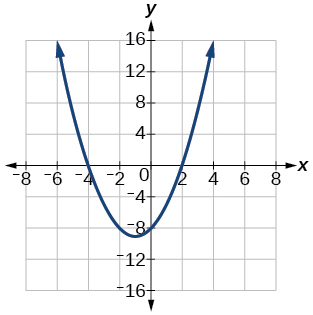Given information about the graph of a quadratic function, find its equation.

Vertex $\text{\hspace{0.17em}}\left(2,0\right)\text{\hspace{0.17em}}$ and point on graph $\text{\hspace{0.17em}}\left(4,12\right).$

Solve the following application problem.

A rectangular field is to be enclosed by fencing. In addition to the enclosing fence, another fence is to divide the field into two parts, running parallel to two sides. If 1,200 feet of fencing is available, find the maximum area that can be enclosed.

60,000 square feet

Find all zeros of the following polynomial functions, noting multiplicities.

$f\left(x\right)={\left(x-3\right)}^{3}\left(3x-1\right){\left(x-1\right)}^{2}$

$f\left(x\right)=2{x}^{6}-12{x}^{5}+18{x}^{4}$

0 with multiplicity 4, 3 with multiplicity 2

Based on the graph, determine the zeros of the function and multiplicities.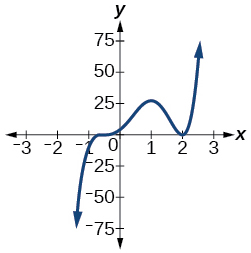Use long division to find the quotient.

$\frac{2{x}^{3}+3x-4}{x+2}$

$2{x}^{2}-4x+11-\frac{26}{x+2}$

Use synthetic division to find the quotient. If the divisor is a factor, write the factored form.

$\frac{{x}^{4}+3{x}^{2}-4}{x-2}$

$\frac{2{x}^{3}+5{x}^{2}-7x-12}{x+3}$

$2{x}^{2}-x-4$ . So factored form is $\text{\hspace{0.17em}}\left(x+3\right)\left(2{x}^{2}-x-4\right)$

Use the Rational Zero Theorem to help you find the zeros of the polynomial functions.

$f\left(x\right)=2{x}^{3}+5{x}^{2}-6x-9$

$f\left(x\right)=4{x}^{4}+8{x}^{3}+21{x}^{2}+17x+4$

$-\frac{1}{2}\text{\hspace{0.17em}}$ (has multiplicity 2), $\text{\hspace{0.17em}}\frac{-1±i\sqrt{15}}{2}\text{\hspace{0.17em}}$

$f\left(x\right)=4{x}^{4}+16{x}^{3}+13{x}^{2}-15x-18$

$f\left(x\right)={x}^{5}+6{x}^{4}+13{x}^{3}+14{x}^{2}+12x+8$

$\text{\hspace{0.17em}}-2\text{\hspace{0.17em}}$ (has multiplicity 3), $\text{\hspace{0.17em}}±i$

Given the following information about a polynomial function, find the function.

It has a double zero at $\text{\hspace{0.17em}}x=3\text{\hspace{0.17em}}$ and zeros at $\text{\hspace{0.17em}}x=1\text{\hspace{0.17em}}$ and $\text{\hspace{0.17em}}x=-2\text{\hspace{0.17em}}$ . Its y -intercept is $\text{\hspace{0.17em}}\left(0,12\right).\text{\hspace{0.17em}}$

It has a zero of multiplicity 3 at $\text{\hspace{0.17em}}x=\frac{1}{2}\text{\hspace{0.17em}}$ and another zero at $\text{\hspace{0.17em}}x=-3\text{\hspace{0.17em}}$ . It contains the point $\text{\hspace{0.17em}}\left(1,8\right).$

$f\left(x\right)=2{\left(2x-1\right)}^{3}\left(x+3\right)$

Use Descartes’ Rule of Signs to determine the possible number of positive and negative solutions.

$8{x}^{3}-21{x}^{2}+6=0$

For the following rational functions, find the intercepts and horizontal and vertical asymptotes, and sketch a graph.

$f\left(x\right)=\frac{x+4}{{x}^{2}-2x-3}$

Intercepts $\text{\hspace{0.17em}}\left(-4,0\right),\text{\hspace{0.17em}}\left(0,-\frac{4}{3}\right)\text{\hspace{0.17em}}$ , Asymptotes .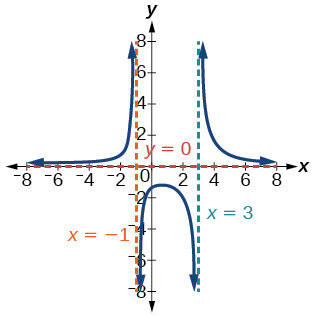$f\left(x\right)=\frac{{x}^{2}+2x-3}{{x}^{2}-4}$

Find the slant asymptote of the rational function.

$f\left(x\right)=\frac{{x}^{2}+3x-3}{x-1}$

$y=x+4$

Find the inverse of the function.

$f\left(x\right)=\sqrt{x-2}+4$

$f\left(x\right)=3{x}^{3}-4$

${f}^{-1}\left(x\right)=\sqrt{\frac{x+4}{3}}$

$f\left(x\right)=\frac{2x+3}{3x-1}$

Find the unknown value.

$\text{\hspace{0.17em}}y\text{\hspace{0.17em}}$ varies inversely as the square of $\text{\hspace{0.17em}}x\text{\hspace{0.17em}}$ and when $\text{\hspace{0.17em}}x=3,\text{\hspace{0.17em}}$ $y=2.\text{\hspace{0.17em}}$ Find $\text{\hspace{0.17em}}y\text{\hspace{0.17em}}$ if $\text{\hspace{0.17em}}x=1.$

$y=18$

$\text{\hspace{0.17em}}y\text{\hspace{0.17em}}$ varies jointly with $\text{\hspace{0.17em}}x\text{\hspace{0.17em}}$ and the cube root of $\text{\hspace{0.17em}}z.\text{\hspace{0.17em}}$ If when $\text{\hspace{0.17em}}x=2\text{\hspace{0.17em}}$ and $\text{\hspace{0.17em}}z=27,\text{\hspace{0.17em}}$ $y=12,\text{\hspace{0.17em}}$ find $\text{\hspace{0.17em}}y\text{\hspace{0.17em}}$ if $\text{\hspace{0.17em}}x=5\text{\hspace{0.17em}}$ and $\text{\hspace{0.17em}}z=8.$

Solve the following application problem.

The distance a body falls varies directly as the square of the time it falls. If an object falls 64 feet in 2 seconds, how long will it take to fall 256 feet?

4 seconds

#### Questions & Answers

prove sin²x+cos²x=3+cos4x
Kiddy Reply
the difference between two signed numbers is -8.if the minued is 5,what is the subtrahend
jeramie Reply
the difference between two signed numbers is -8.if the minuend is 5.what is the subtrahend
jeramie
what are odd numbers
micheal Reply
numbers that leave a remainder when divided by 2
Thorben
1,3,5,7,... 99,...867
Thorben
7%2=1, 679%2=1, 866245%2=1
Thorben
the third and the seventh terms of a G.P are 81 and 16, find the first and fifth terms.
Suleiman Reply
if a=3, b =4 and c=5 find the six trigonometric value sin
Martin Reply
ask
Ans
pls how do I factorize x⁴+x³-7x²-x+6=0
Gift Reply
in a function the input value is called
Rimsha Reply
how do I test for values on the number line
Modesta Reply
if a=4 b=4 then a+b=
Rimsha Reply
a+b+2ab
Kin
commulative principle
DIOSDADO
a+b= 4+4=8
Mimi
If a=4 and b=4 then we add the value of a and b i.e a+b=4+4=8.
Tariq
what are examples of natural number
sani Reply
an equation for the line that goes through the point (-1,12) and has a slope of 2,3
Katheryn Reply
3y=-9x+25
Ishaq
show that the set of natural numberdoes not from agroup with addition or multiplication butit forms aseni group with respect toaaddition as well as multiplication
Komal Reply
x^20+x^15+x^10+x^5/x^2+1
Urmila Reply
evaluate each algebraic expression. 2x+×_2 if ×=5
Sarch Reply
if the ratio of the root of ax+bx+c =0, show that (m+1)^2 ac =b^2m
Awe Reply

### Read also:

#### Get Jobilize Job Search Mobile App in your pocket Now!

Source:  OpenStax, Algebra and trigonometry. OpenStax CNX. Nov 14, 2016 Download for free at https://legacy.cnx.org/content/col11758/1.6
Google Play and the Google Play logo are trademarks of Google Inc.

Notification Switch

Would you like to follow the 'Algebra and trigonometry' conversation and receive update notifications?ByByBy OpenStaxBy OpenStaxBy Yasser IbrahimBy OpenStaxBy Madison ChristianBy OpenStaxBy Vanessa SoledadBy Sandhills MLTBy Anonymous UserBy OpenStax